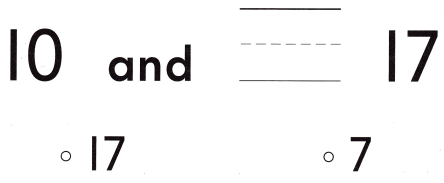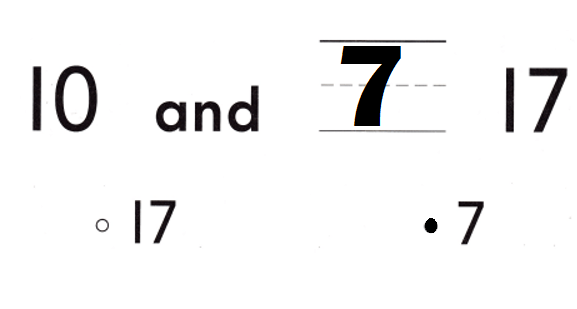# Texas Go Math Kindergarten Lesson 8.4 Answer Key Count and Write 18 and 19

Refer to our Texas Go Math Kindergarten Answer Key Pdf to score good marks in the exams. Test yourself by practicing the problems from Texas Go Math Kindergarten Lesson 8.4 Answer Key Count and Write 18 and 19.

## Texas Go Math Kindergarten Lesson 8.4 Answer Key Count and Write 18 and 19

Explore

DIRECTIONS: Count and tell how many. Trace and write the numbers and the words.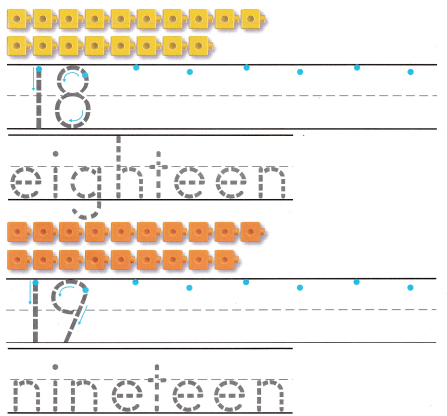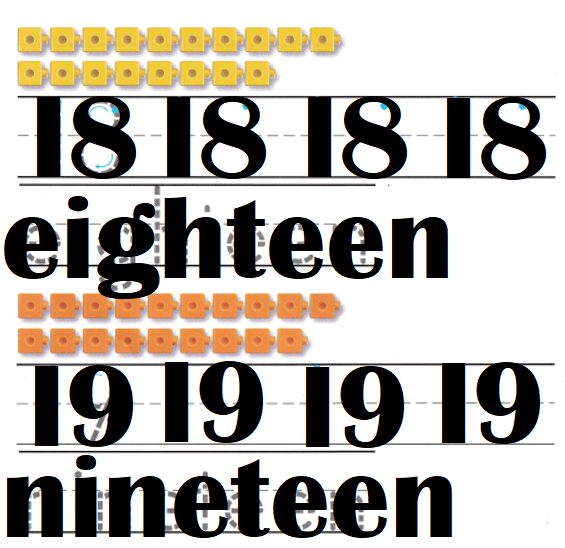Explanation:
Number of yellow cubes = 18 or Eighteen.
Number of oranges cubes = 19 or Nineteen.

Share and Show

DIRECTIONS: 1. Count and tell how many. Practice writing the numbers. 2. Count and tell how many. Write the number. 3. Look at the ten frames in Exercise 2. Write how many green squares. Write how many blue squares. Write how many in all.

Question 1.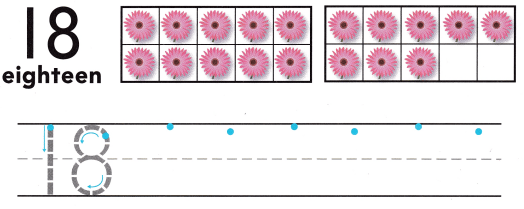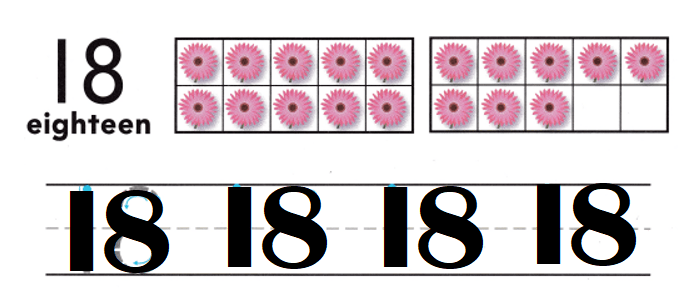Explanation:
Number of flowers = 10 + 8 = 18 or Eighteen.

Question 2.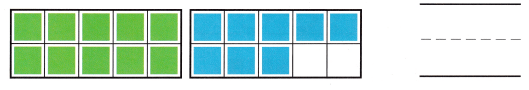Explanation:
Number of green cubes = 10.
Number of blue cubes = 8.
Total number of cubes = Number of green cubes + Number of blue cubes
= 10 + 8
= 18 or Eighteen.

Question 3.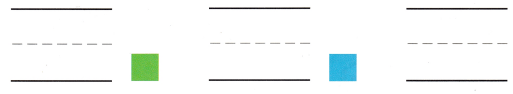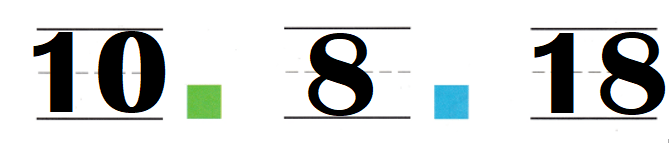Explanation:
Number of green cubes = 10.
Number of blue cubes = 8.
Total number of cubes = Number of green cubes + Number of blue cubes
= 10 + 8
= 18 or Eighteen.

DIRECTIONS: 4. Count and tell how many. Practice writing the numbers. 5. Count and tell how many. Write the number. 6. Look at the ten frames in Exercise 5. Write how many blue squares. Write how many red squares. Write how many in all.

Question 4.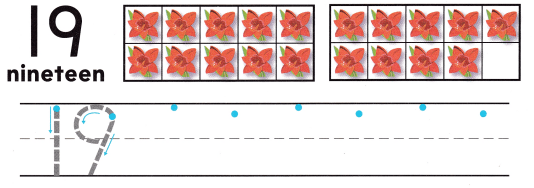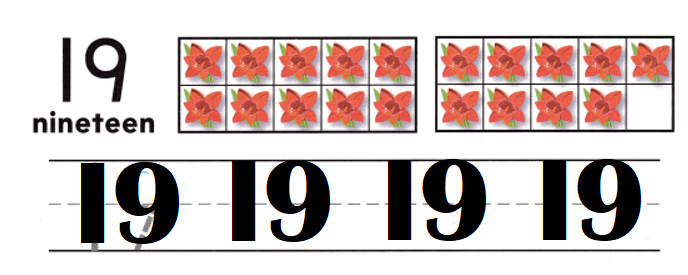Explanation:
Number of flowers = 10 + 9 = 19 or Nineteen.

Question 5.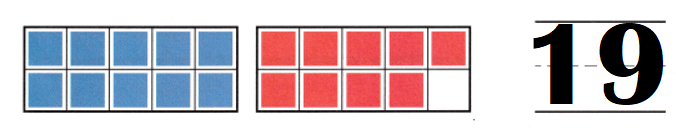Explanation:
Number of blue cubes = 10.
Number of red cubes = 9.
Total number of cubes = Number of blue cubes + Number of red cubes
= 10 + 9
= 19 or Nineteen.

Question 6.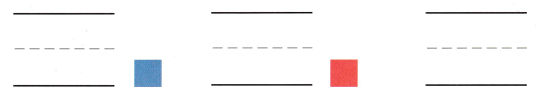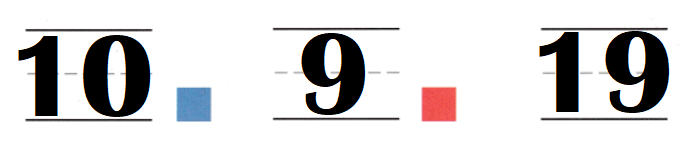Explanation:
Number of blue cubes = 10.
Number of red cubes = 9.
Total number of cubes = Number of blue cubes + Number of red cubes
= 10 + 9
= 19 or Nineteen.

HOME ACTIVITY • Ask your child to count and write the number for a set of 18 or 19 objects, such as macaroni pieces or buttons.
Number of buttons = 19 or Nineteen.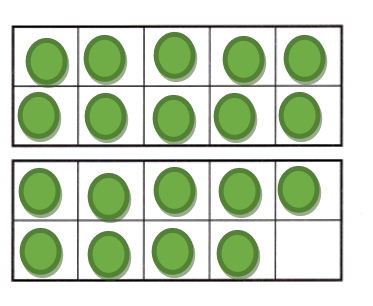Explanation:
My kid took buttons and counted and told.
Number of buttons = 18 or Eighteen.DIRECTIONS: 7. Jonah has 10 purple flowers. Kaiya has 8 red flowers. How many flowers do they have in all? Write the number. 8. Draw a set of 19 objects. Circle 10 of the objects. How many more objects are there? Write the number. 9. Choose the correct answer. What number goes in the blank?

Problem Solving
Question 7.Explanation:
Number of purple flowers = 10.
Number of red flowers = 8.
Total number of flowers = Number of purple flowers + Number of red flowers
= 10 + 8
= 18 or Eighteen.

Question 8.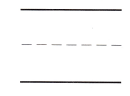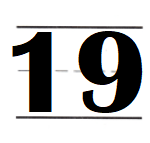Explanation:
Number of counters = 10 + 9 = 19 or Nineteen.Question 9.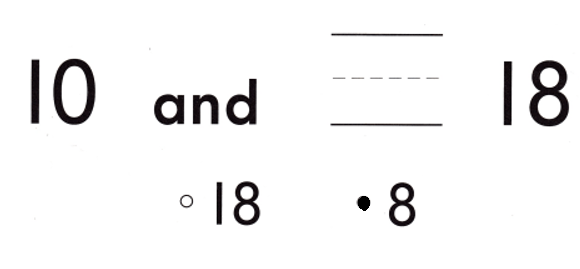Explanation:
10 + 8 = 18 or Eighteen.

### Texas Go Math Kindergarten Lesson 8.4 Homework and Practice Answer Key

DIRECTIONS: 1-2. Count and tell how many. Write the numbers.

Question 1.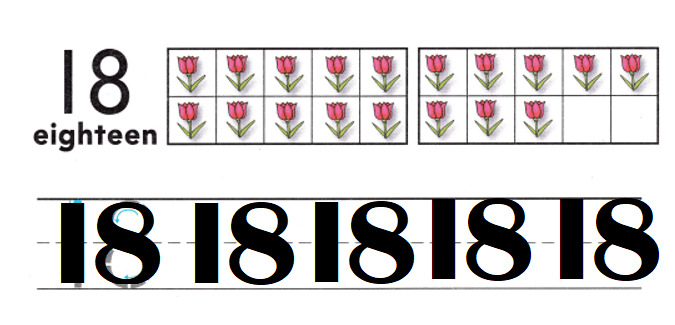Explanation:
Number of flowers = 10 + 8 = 18 or Eighteen.

Question 2.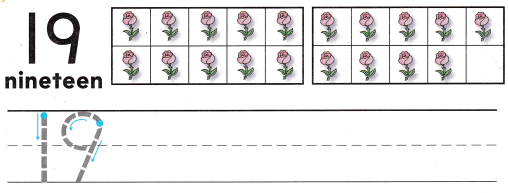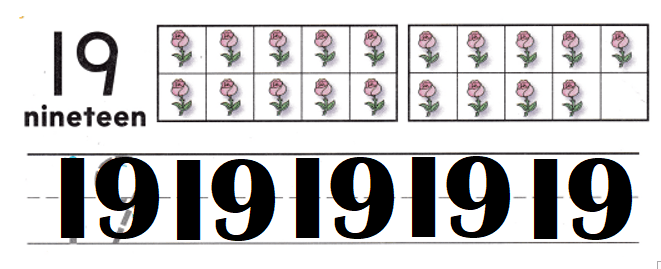Explanation:
Number of flowers = 19 or Nineteen.

DIRECTIONS: 3-5. Choose the correct answer. What number goes in the blank?

Lesson Check
Question 3.Explanation:
10 + 9 = 19 or Nineteen.

Question 4.Explanation:
10 + 8 = 18 or Eighteen.

Question 5.